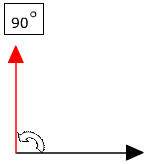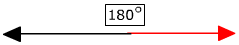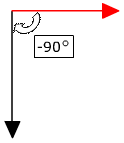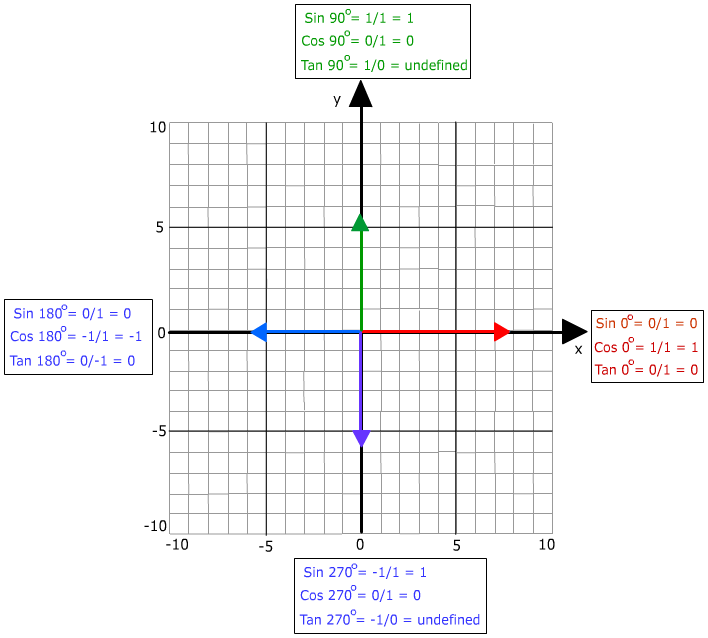A Quadrantal Angle is an angle in standard position with terminal side on the x-axis or y-axis. Some examples are the angles located at 0°, 90°, 180°, 270°, 360°, 450°, ... as well as -90°, -180°, -270°, -360°The Trigonometric functions of the Quadrantal angles:If the three basis trig functions (sine, cosine and tangent) are known then the secant, cosecant and cotangent can be found by using reciprocals. For instance cot 0° is the reciprocal of tan 0° therefore cot 0° = 1/0 = undefined.

Quandrantal angles are easy to find because they will always have the initial side and the terminal side on an axis. Remember that the angles can be positive or negative depending on whether the direction is clockwise (negative) or counterclockwise (positive).

 Related Links: Math Trigonometry Reference Angles Coterminal Angles